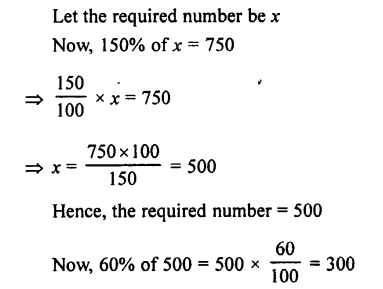## Selina Concise Mathematics class 7 ICSE Solutions – Percent and Percentage

APlusTopper.com provides step by step solutions for Selina Concise ICSE Solutions for Class 7 Mathematics. You can download the Selina Concise Mathematics ICSE Solutions for Class 7 with Free PDF download option. Selina Publishers Concise Mathematics for Class 7 ICSE Solutions all questions are solved and explained by expert mathematic teachers as per ICSE board guidelines.

POINTS TO REMEMBER

1. The cent means hundred. Therefore percent means after hundred and notation % is used for it.
2. To express an ordinary given statement as percent.
(i) Express the given statement as a fraction.
(ii) Convert this fraction into an equivalent fraction with denominator 100.
Therefore to express a fraction or a decimal as percent, multiply it by 100.
3. To Express-One quantity as a percent of the other.
(i) If necessary, convert with the quantitities into the same units.
(ii) From the fraction with the number to be compared as numerator and the number with which it is to be compared as denominator.
(iii) Multiply the fraction obtained by 100 and at the same time write the percent sign (%).

EXERCISE 8 (A)

Question 1.
Express each of the following as percent :Solution :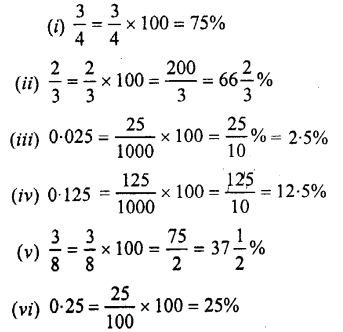Question 2.
Express the following percentages as fractions and as decimal numbers :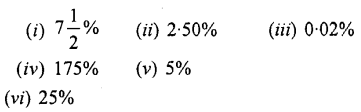Solution :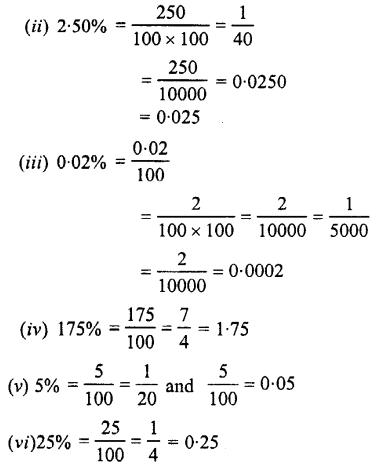Question 3.
What percent is :
(i) 16 hours of 2 days ?
(ii) 40 paisa of Rs. 2 ?
(iii) 25 cm of 4 metres
(iv) 600 gm of 5 kg ?

Solution :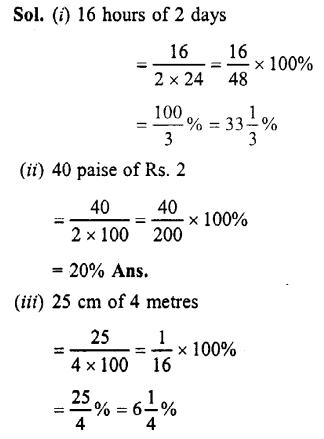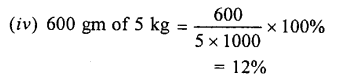Question 4.
Find the value of:Solution :Question 5.
In a class of 60 children, 30% are girls. How many boys are there ?

Solution :
Total children = 60,
Girls = 30%
∴Total girls = 30% of 60 = 60 x $$\frac { 30 }{ 100 }$$= 18
∴ No. of boys = 60 – 18 = 42

Question 6.
In an election, two candidates A and B contested. A got 60% of the votes. The total votes polled were 8000. How many votes did each get ?

Solution :
Total number of votes polled = 8000
A got 60% of the votes
A got total votes = 60% of 8000 = 8000 x $$\frac { 60 }{ 100 }$$ = 4800
∴ B got total votes = 8000 – 4800 = 3200

Question 7.
A person saves 12% of his salary every month. If his salary is ₹2,500, find his expenditure.

Solution :
Total salary = ₹2500
Saving = 12% of the salary
∴ Total savings = 12% of ₹2500
= ₹2500 x$$\frac { 12 }{ 100 }$$ = ₹300
∴Total expenditure = ₹2500 – ₹300 = ₹2200

Question 8.
Seeta got 75% marks out of a total of 800. How many marks did she lose ?

Solution :
Total marks = 800
Marks Seeta got = 75% of total marks
∴ Total marks Seeta got = 75% of 800
= 800 x $$\frac { 75 }{ 100 }$$ = 600
∴ Marks Seeta lose = 800 – 600 = 200

Question 9.
A shop worth ₹25,000 was insured for 95% of its value. How much would the owner get in case of any mishappening ?

Solution :
Value of shop =₹25,000
Insured amount = 95% of total value
=95% of ₹25,000
= ₹25,000 x $$\frac { 95 }{ 100 }$$
= ₹ 23,750

Question 10.
A class has 30 boys and 25 girls. What is the percentage of boys in the class ?

Solution :
No. of boys = 30
No. of girls = 25
Total number of children = 30 + 25 = 55
∴Percentage of boys in the class
= $$\frac { 30 }{ 55 }$$ x 100
= $$\frac { 600 }{ 11 }$$=54$$\frac { 6 }{ 11 }$$ %

Question 11.
Express :
(i) 3 $$\frac { 2 }{ 5 }$$ as a percent
(ii) 0.0075 as percent
(iii) 3 : 20 as percent
(iv) 60 cm as percent of 1 m 25 cm
(v) 9 hours as a percent of 4 days.

Solution :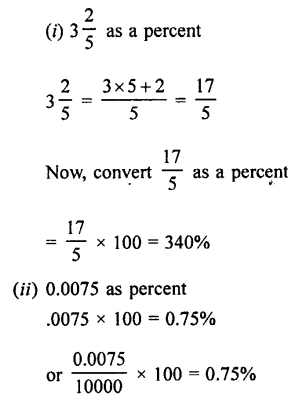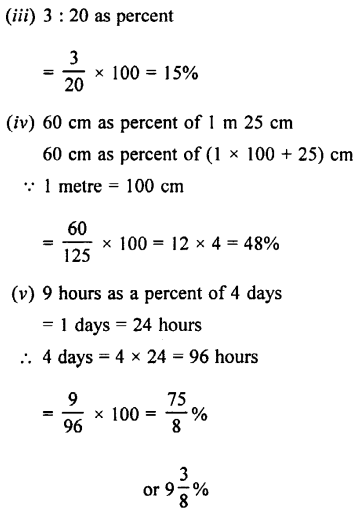Question 12.
(i) Find 2% of 2 hours 30 min.
(ii) What percent of 12 kg is 725 gm?

Solution :EXERCISE 8 (B)

Question 1.
Deepak bought a basket of mangoes containing 250 mangoes 12% of these were found to be rotten. Of the remaining, 10% got crushed. How many mangoes were in good condition ?

Solution :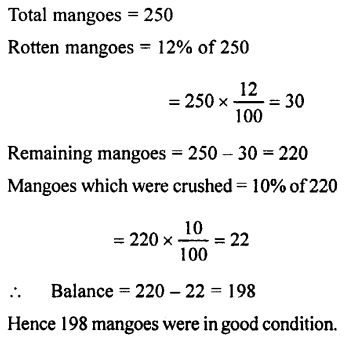Question 2.
In a Maths Quiz of 60 questions, Chandra got 90% correct answers and Ram got 80% correct answers. How many correct answers did each give ?

Solution :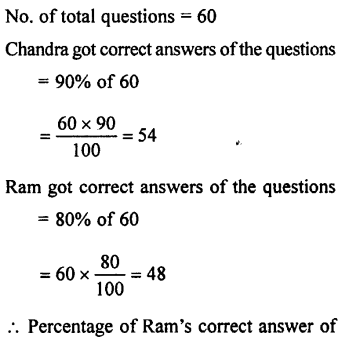Question 3.
In an examination, the maximum marks are 900. A student gets 33% of the maximum marks and fails by 45 marks. What is the passing mark ? Also, find the pass percentage.

Solution :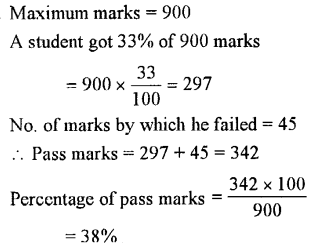Question 4.
In a train, 15% people travel in first class, 35% travel in second class. The balance travel in the A.C. class ? Calculate the percentage of A.C. class travellers ?

Solution :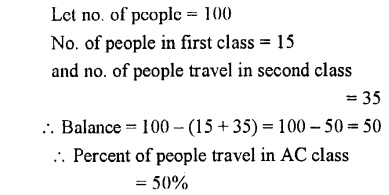Question 5.
A boy eats 25% of the cake and gives away 35% of it to his friends. What percent of the cake is still left with him ?

Solution :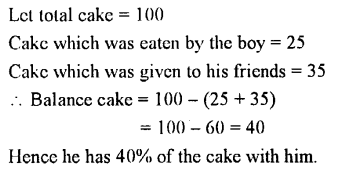Question 6.
What is the percentage of vowels in the English alphabet ?

Solution :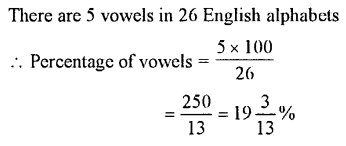Question 7.Solution :Question 8.
The money spent on the repairs of a house was 1% of its value. If the repair, costs Rs. 5,000, find the cost of the house.

Solution :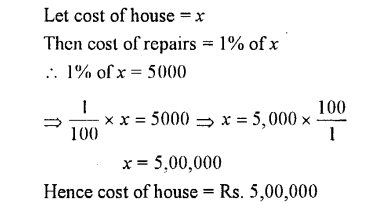Question 9.
In a school out of300 students, 70% are girls and 30% are boys. If 30 girls leave and no new boy is admitted, what is the new percentage of girls in the school ?

Solution :Question 10.
Kumar bought a transistor for Rs. 960. He paid 12 $$\frac { 1 }{ 2 }$$ % cash money. The rest he agreed to pay in 12 equal monthly instalments. How much will he pay each month ?

Solution :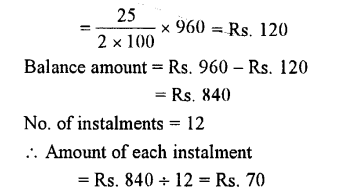Question 11.
An ore contains 20% zinc. How many kg of ore will be required to get 45 kg of zinc ?

Solution :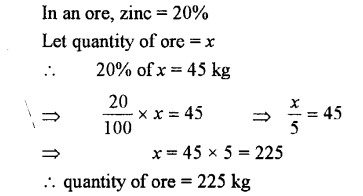EXERCISE 8 (C)

Question 1.
The salary of a man is increased from Rs. 600 per month to Rs. 850 per month. Express the increase in salary as percent.

Solution :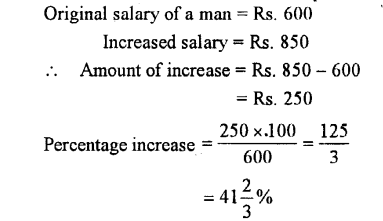Question 2.
Increase :
(i) 60 by 5%
(ii) 20 by 15%
(iii) 48 by 121 %
(iv) 80 by 140%
(v) 1000 by 3.5%

Solution :Question 3.
Decrease :
(i)80 by 20%
(ii) 300 by 10%
(iii) 50 by 12.5%

Solution :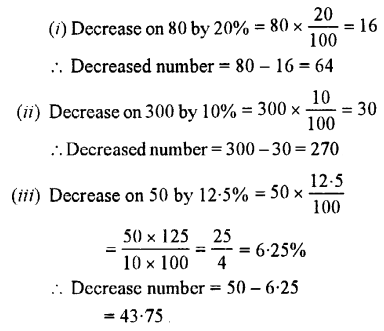Question 4.
What number :
(i) When increased by 10% becomes 88 ?
(ii) When increased by 15% becomes 230 ?
(iii) When decreased by 15% becomes 170 ?
(iv) When decreased by 40% becomes 480 ?
(v) When increased by 100% becomes 100 ?
(vi) When decreased by 50% becomes 50 ?

Solution :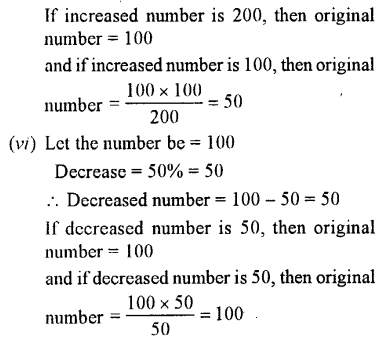Question 5.
The price of a car is lowered by 20% to Rs. 40,000. What was the original price ? Also, find the reduction in price.

Solution :
Let original price of the car = Rs. 100
Reduction = 20%’ = Rs. 20
∴ Reduced price = Rs. 100 – 20 = Rs. 80
If reduced price is Rs. 80, then original price = Rs. 100
and if reduced price is Rs. 40,000 then original price = $$\frac { Rs.100 x 40000 }{ 80 }$$
= Rs. 50,000
and reduction = Rs. 50000 – Rs. 40000
= Rs. 10,000

Question 6.
If the price of an article is increased by 25%, The increase is Rs. 10. Find the new price.

Solution :
Let the price of an article = Rs. 100
Increase = 25%
∴Increase = Rs. 25
If an increased price = Rs. 100 + 25 = Rs. 125
If increase is Rs. 25 then new price = Rs. 125
and if increase is Rs. 10, then new price = Rs. $$\frac { 125 x 10 }{ 25 }$$
= Rs. 50

Question 7.
If the price of an article is reduced by 10%, the reduction is Rs. 40. What is the old price ?

Solution :
Let the original (old) price = Rs. 100
Reduction = 10% = Rs. 10
∴If reduction is Rs. 10, then old price = Rs. 100
and if reduction is Rs. 40, then old price = Rs.$$\frac { 100 x 40 }{ 10 }$$ = Rs. 400

Question 8.
The price of a chair is reduced by 25%. What is the ratio of:
(i) Change in price to the old price.
(ii) Old price to the new price.

Solution :
Let old (original) price of a chair = Rs. 100
Reduction = 25% = Rs. 25
∴Reduced price = Rs. 100 – Rs. 25 = Rs. 75
(i) Ratio between change in price and old price = 25 : 100
= 1:4 (Dividing by 25)
(ii) Ratio between old price and new price = 100 : 75
= 4:3 (Dividing by 25)

Question 9.
If x is 20% less than y, find :Solution :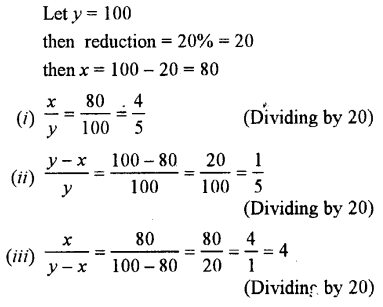Question 10.
If x is 30% more than y; find :Solution :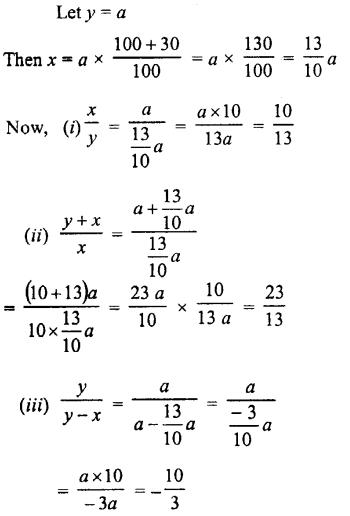Question 11.
The weight of a machine is 40 kg. By mistake it was weighed as 40.8 kg. Find the error percent.

Solution :Question 12.
From a cask, containing 450 litres of petrol, 8% of the petrol was lost by leakage and evaporation. How many litres of petrol was left in the cask ?

Solution :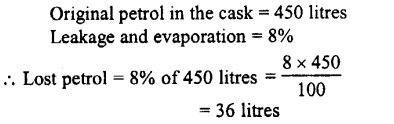Question 13.
An alloy consists of 13 parts of copper, 7 parts of zinc and 5 parts of nickel. What is the percentage of each metal in the alloy?

Solution :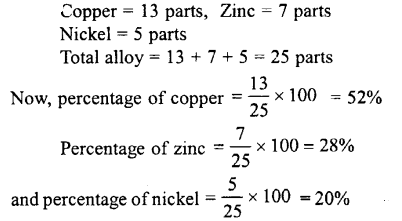Question 14.
In an examination, first division marks are 60%. A student secures 538 marks and misses the first division by 2 marks. Find the total marks of the examination.

Solution :Question 15.
Out of 1200 pupils in a school, 900 are boys and the rest are girls. If 20% of the boys and 30% of the girls wear spectacles, find :
(i) how many pupils in all, wear spectacles ?
(ii) what percent of the total number of pupils wear spectacles ?

Solution :Question 16.
Out of 25 identical bulbs, 17 are red, 3 are black and the remaining are yellow. Find the difference between the numbers of red and yellow bulbs and express this difference as percent.

Solution :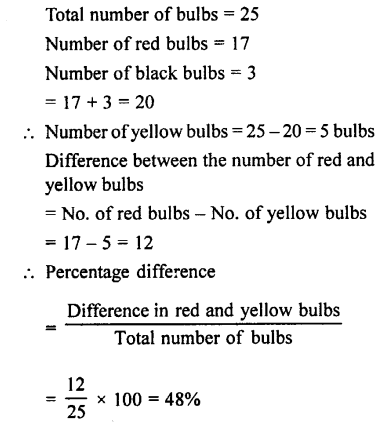Question 17.
A number first increases by 20% and then decreases by 20%. Find the percentage increase or decrease on the whole.

Solution :Question 18.
A number is first decreased by 40% and then again decreased by 60%. Find the percentage increase or decrease on the whole.

Solution :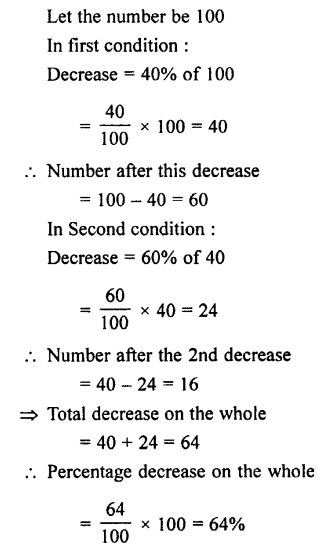Question 19.
If 150% of a number is 750, find 60% of this number.

Solution :